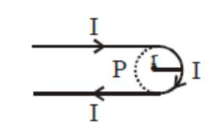# A hairpin like shape as shown in figure is made by bending a long current carrying wire.

Question:

A hairpin like shape as shown in figure is made by bending a long current carrying wire. What is the magnitude of a magnetic field at point $\mathrm{P}$ which lies on the centre of the semicircle?1. (1) $\frac{\mu_{0} \mathrm{I}}{4 \pi \mathrm{r}}(2-\pi)$

2. (2) $\frac{\mu_{0} \mathrm{I}}{4 \pi \mathrm{r}}(2+\pi)$

3. (3) $\frac{\mu_{0} \mathrm{I}}{2 \pi_{\mathrm{r}}}(2+\pi)$

4. (4) $\frac{\mu_{0} \mathrm{I}}{2 \pi_{\mathrm{r}}}(2-\pi)$

Correct Option: , 2

Solution:

(2)

$B=2 \times B_{\text {st.wire }}+B_{\text {loop }}$

$\mathrm{B}=2 \times \frac{\mu_{0} \mathrm{i}}{4 \pi \mathrm{r}}+\frac{\mu_{0} \mathrm{i}}{2 \mathrm{r}}\left(\frac{\pi}{2 \pi}\right)$

$\mathrm{B}=\frac{\mu_{0} \mathrm{i}}{4 \pi \mathrm{r}}(2+\pi)$